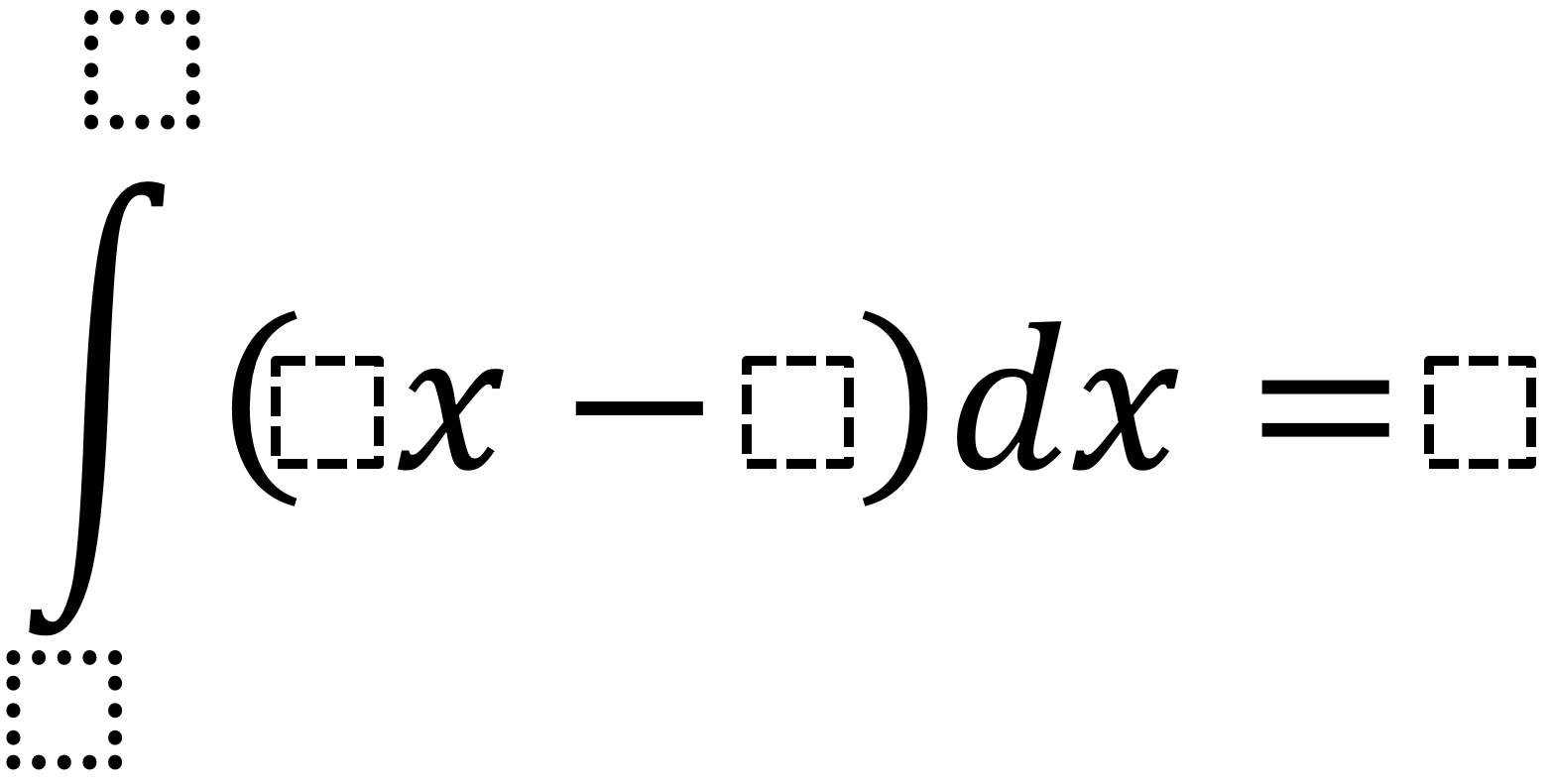Home > Calculus > Definite Integral

Definite Integral

Directions: Use the integers 1-9, without repetition, to fill in the empty spaces so that the equation with the definite integral is true.Hint

The integral represents the area under the curve. What does this look like on a graph?

Integral from 2 to 4 of 3x-5 equals 8. (This would have to be formatted as an equation).

Source: Daniel Torres-Rangel

Derivative of Trig Functions 2

Directions: Fill in the boxes below using the digits 1 to 6, at most one …

1.•